알림2021 Efficiency evaluation of the microgrid for selection of common bus

Conventionally, nuclear and thermal power plants were used to generate power and provide electricity to the residential, commercial ,and industrial loads at long distances. However, due to the carbon emissions from these power plants and increased energy demand, alternative environmentally friendly and cost-free resources are encouraged. Therefore, renewable energy resources (RERs) such as wind, photovoltaic (PV) ,and fuel cell are being used nowadays. They constitute a smaller grid (known as microgrids) that comprises RERs, energy storage systems (ESS) ,and loads. Furthermore, they are connected to the utility grid. These microgrids are capable of generating, managing, and supplying local loads using renewable energy sources. All the components in the microgrid are connected at the common bus through suitable conversion stages. The RER-based microgrid with DC and AC common bus is shown in Fig. 1.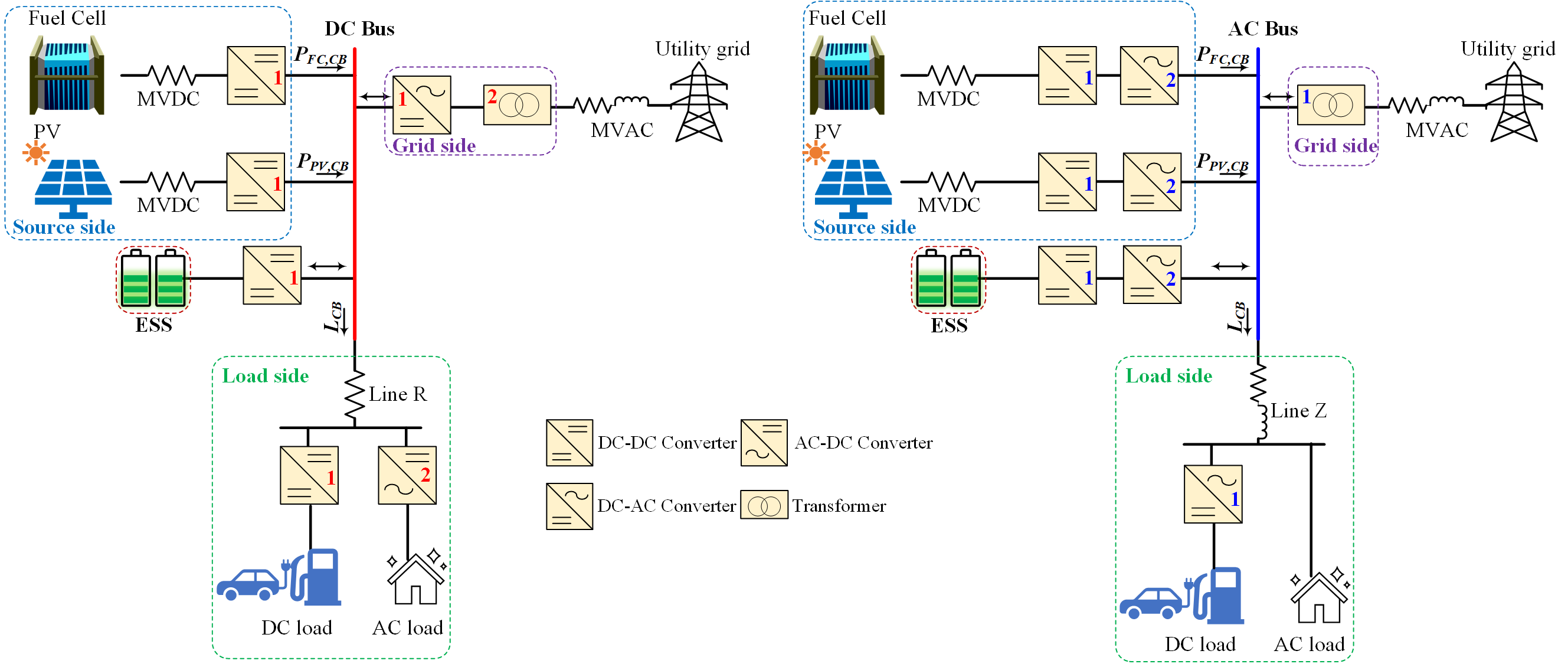(a)                                                                                                                                                 (b)

Fig. 1. Microgrid block diagram with: (a) DC common bus, (b) AC common bus. (Converter’s index represents the converter number on a specific side).

As shown in Fig. 1(a) for DC common bus, RERs, ESS ,and DC load are connected via DC-DC converter whereas utility grid and AC load are connected via DC-AC converter. Likewise, for AC common bus in Fig. 1(b), each component is connected through a suitable conversion stage. The nature of the common bus defines the number of conversion stages, hence, affecting the total power loss in the microgrid. Therefore, a feasibility analysis of the common bus type is required at the early design stage to improve the design performance. In this study, we evaluated the feasibility of the common bus type in terms of efficiency of the microgrid by using the copula function-based efficiency curves of the converters.

The first step in efficiency evaluation is to determine the percentage loading of the converters. To calculate the percentage loading, the instantaneous load on all the converters is evaluated in accordance with the load sharing scheme explained below:

Idle mode:

During the idle mode, generation and load demand are equal. Therefore, the utility grid and ESS converters remain in idle state. Percentage loading on other converters is found according to load demand and generation in the microgrid.

Excess power mode:

During the excess power mode, generation is greater than load demand. Excess power is used to charge the ESS and if ESS is fully charged, excess power is sent to the utility grid.

Deficit power mode:

For the deficit power mode, generation is less than load demand. ESS is employed to provide the deficit power. However, if ESS is fully depleted, the utility grid charges the ESS and supplies power to the load demand. Following this scheme, the percentage loading on all the converters is determined.

Having obtained the percentage loading of all the converters, the efficiency of each converter is obtained using the copula function-based efficiency curve model of the converter. The procedure to obtain this model is shown in Fig. 2.  As it can be seen in Fig. 2(a), the efficiency of the converter is a function of percentage loading. This efficiency curve is obtained for each converter. During the second phase, the probability distribution functions of efficiency and percentage loading are obtained using bootstrap parametric estimation, as shown in Fig. 2(b). In order to obtain the best-fitted probability distributions, the goodness of fit test is performed using Kolmogorov Smirnov test. The first ranked distribution is considered as best-fitted distribution. During the third phase, copula fitting is performed as shown in Fig. 2(c). The best-fitted copula according to the Akaike Information criteria is selected. In this study, we have used the copula function to model the efficiency curves of the converters because they are capable of characterizing the nonlinear and non-constant relationship between variables. Using this model, the efficiency of the converters against each percentage loading can be obtained.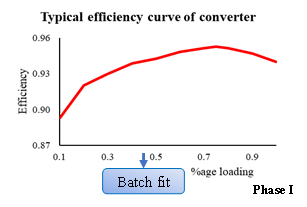(a)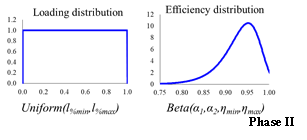(b)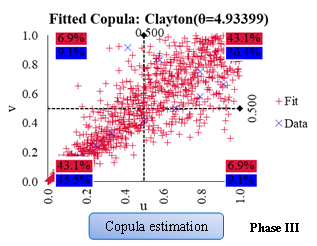(c)

Fig. 2. Procedure for copula estimation: (a) Efficiency curve of the converter, (b) Probability distributions for η and l%, (c) Estimated copula.

During the next step, total power loss inside the microgrid is determined. It is the sum of power loss in all the converters and distribution lines. Having obtained the total power loss, the efficiency of the microgrid can be obtained. We evaluated the efficiency of microgrid shown in Fig. 1 for the following PV generation and load demand scenarios.

PV generation scenarios

1. Cold & Sunny
2. Cold & Cloudy
3. Warm & Humid
4. Hot & Dry

1. Weekend
2. Weekdays

Furthermore, we performed two case studies, first with the DC load only and second with both types of loads in the microgrid.

Results for efficiency evaluation in the case of DC load only for all scenarios are shown in Fig. 3. As it can be seen, efficiency varies depending upon the percentage loading for each scenario. Similarly, Fig. 4 presents the efficiency of the microgrid for all scenarios when both types of loads are present. In this case, the efficiency of AC common bus is improved a little bit.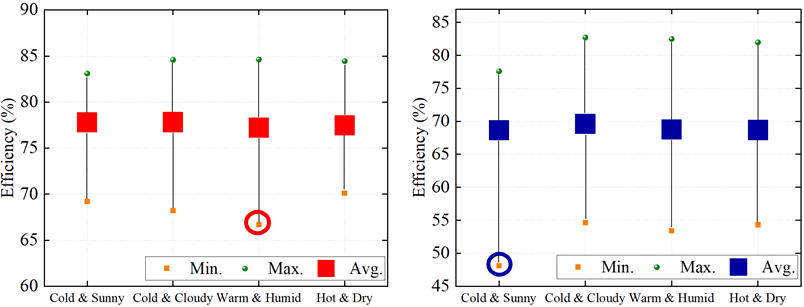(a)                                                                        (b)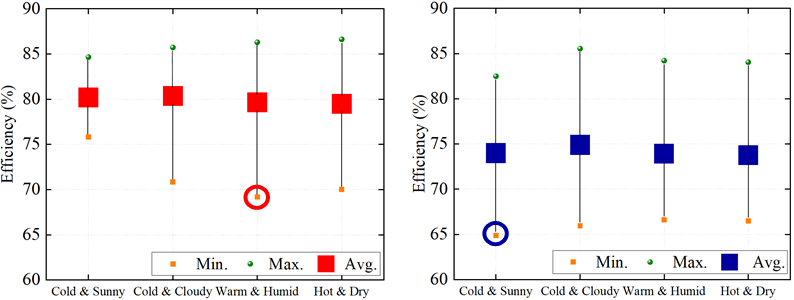(c)                                                                        (d)

Fig. 3. Efficiency of the microgrid for DC load only: (a) Weekday load (DC common bus), (b) Weekday load (AC common bus), (c) Weekend load (DC common bus), (d) Weekend load (AC common bus).(a)                                                                      (b)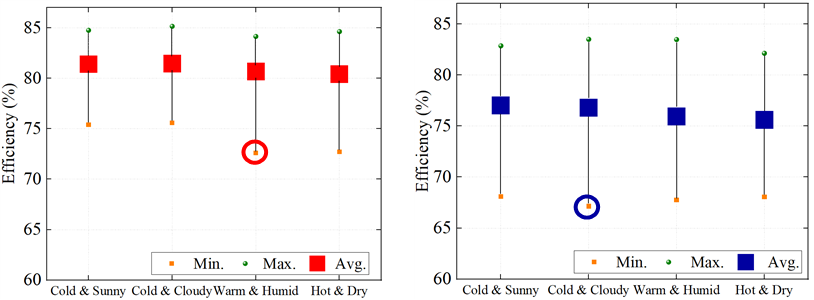(c)                                                                      (d)

Fig. 4. Efficiency of the microgrid for DC +AC load: (a) Weekday load (DC common bus), (b) Weekday load (AC common bus), (c) Weekend load (DC common bus), (d) Weekend load (AC common bus).

In order to select the most feasible common bus type, we calculated the weighted average efficiency for both DC and AC common bus types. The results are shown in Fig. 5. The positive number shows the dominance of DC common bus over its AC counterpart. Therefore, DC common bus is the most feasible common bus type in this study. Moreover, it is concluded that DC common bus is more advantageous in the case of DC load only. As the trend is shifting towards DC, the DC common bus would offer more advantages in the future.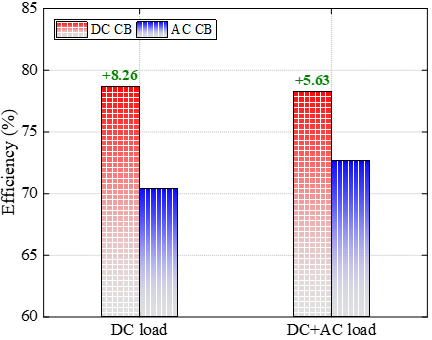Fig. 5. Selection of the most feasible common bus type.